# Lattice (group)

Not to be confused with the partially ordered set, Lattice (order). For other related uses, see Lattice (disambiguation).A lattice in the Euclidean plane.

In mathematics, especially in geometry and group theory, a lattice inis a subgroup ofwhich is isomorphic to, and which spans the real vector space. In other words, for any basis of, the subgroup of all linear combinations with integer coefficients of the basis vectors forms a lattice. A lattice may be viewed as a regular tiling of a space by a primitive cell.

Lattices have many significant applications in pure mathematics, particularly in connection to Lie algebras, number theory and group theory. They also arise in applied mathematics in connection with coding theory, in cryptography because of conjectured computational hardness of several lattice problems, and are used in various ways in the physical sciences. For instance, in materials science and solid-state physics, a lattice is a synonym for the "frame work" of a crystalline structure, a 3-dimensional array of regularly spaced points coinciding in special cases with the atom or molecule positions in a crystal. More generally, lattice models are studied in physics, often by the techniques of computational physics.

## Symmetry considerations and examples

A lattice is the symmetry group of discrete translational symmetry in n directions. A pattern with this lattice of translational symmetry cannot have more, but may have less symmetry than the lattice itself. As a group (dropping its geometric structure) a lattice is a finitely-generated free abelian group, and thus isomorphic to.

A lattice in the sense of a 3-dimensional array of regularly spaced points coinciding with e.g. the atom or molecule positions in a crystal, or more generally, the orbit of a group action under translational symmetry, is a translate of the translation lattice: a coset, which need not contain the origin, and therefore need not be a lattice in the previous sense.

A simple example of a lattice inis the subgroup. More complicated examples include the E8 lattice, which is a lattice in, and the Leech lattice in. The period lattice inis central to the study of elliptic functions, developed in nineteenth century mathematics; it generalises to higher dimensions in the theory of abelian functions. Lattices called root lattices are important in the theory of simple Lie algebras; for example, the E8 lattice is related to a Lie algebra that goes by the same name.

## Dividing space according to a lattice

A typical latticeinthus has the formwhere {v1, ..., vn} is a basis for. Different bases can generate the same lattice, but the absolute value of the determinant of the vectors vi is uniquely determined by Λ, and is denoted by d(Λ). If one thinks of a lattice as dividing the whole ofinto equal polyhedra (copies of an n-dimensional parallelepiped, known as the fundamental region of the lattice), then d(Λ) is equal to the n-dimensional volume of this polyhedron. This is why d(Λ) is sometimes called the covolume of the lattice. If this equals 1, the lattice is called unimodular.

## Lattice points in convex sets

Minkowski's theorem relates the number d(Λ) and the volume of a symmetric convex set S to the number of lattice points contained in S. The number of lattice points contained in a polytope all of whose vertices are elements of the lattice is described by the polytope's Ehrhart polynomial. Formulas for some of the coefficients of this polynomial involve d(Λ) as well.

## Computing with lattices

Lattice basis reduction is the problem of finding a short and nearly orthogonal lattice basis. The Lenstra-Lenstra-Lovász lattice basis reduction algorithm (LLL) approximates such a lattice basis in polynomial time; it has found numerous applications, particularly in public-key cryptography.

## Lattices in two dimensions: detailed discussion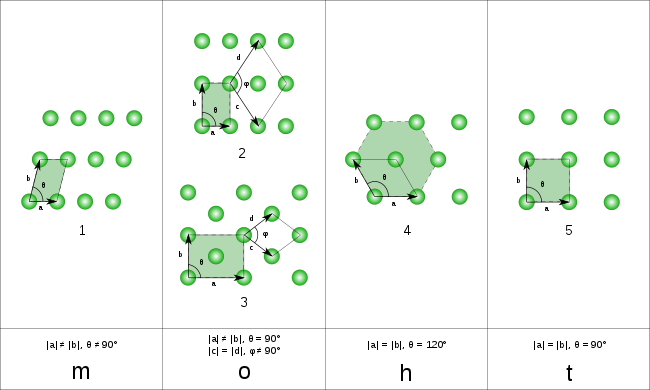Five lattices in the Euclidean plane

There are five 2D lattice types as given by the crystallographic restriction theorem. Below, the wallpaper group of the lattice is given in IUC notation, Orbifold notation, and Coxeter notation, along with a wallpaper diagram showing the symmetry domains. Note that a pattern with this lattice of translational symmetry cannot have more, but may have less symmetry than the lattice itself. A full list of subgroups is available. For example below the hexagonal/triangular lattice is given twice, with full 6-fold and a half 3-fold reflectional symmetry. If the symmetry group of a pattern contains an n-fold rotation then the lattice has n-fold symmetry for even n and 2n-fold for odd n.

cmm, (2*22), [,2+,] p4m, (*442), [4,4] p6m, (*632), [6,3]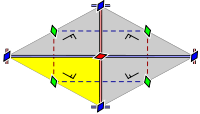rhombic lattice
also centered rectangular lattice
isosceles triangular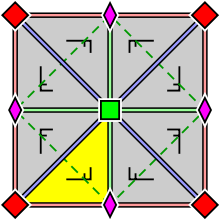square lattice
right isosceles triangular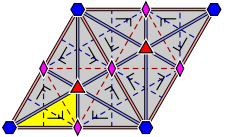hexagonal lattice
(equilateral triangular lattice)
pmm, *2222, [,2,] p2, 2222, [,2,]+ p3m1, (*333), [3]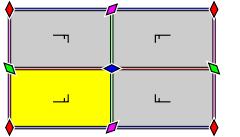rectangular lattice
also centered rhombic lattice
right triangular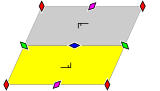parallelogrammic lattice
also oblique lattice
scalene triangular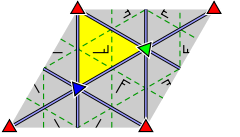equilateral triangular lattice
(hexagonal lattice)

For the classification of a given lattice, start with one point and take a nearest second point. For the third point, not on the same line, consider its distances to both points. Among the points for which the smaller of these two distances is least, choose a point for which the larger of the two is least. (Not logically equivalent but in the case of lattices giving the same result is just "Choose a point for which the larger of the two is least".)

The five cases correspond to the triangle being equilateral, right isosceles, right, isosceles, and scalene. In a rhombic lattice, the shortest distance may either be a diagonal or a side of the rhombus, i.e., the line segment connecting the first two points may or may not be one of the equal sides of the isosceles triangle. This depends on the smaller angle of the rhombus being less than 60° or between 60° and 90°.

The general case is known as a period lattice. If the vectors p and q generate the lattice, instead of p and q we can also take p and p-q, etc. In general in 2D, we can take a p + b q and c p + d q for integers a,b, c and d such that ad-bc is 1 or -1. This ensures that p and q themselves are integer linear combinations of the other two vectors. Each pair p, q defines a parallelogram, all with the same area, the magnitude of the cross product. One parallelogram fully defines the whole object. Without further symmetry, this parallelogram is a fundamental parallelogram.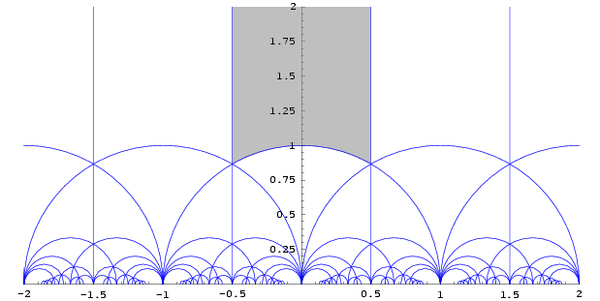The vectors p and q can be represented by complex numbers. Up to size and orientation, a pair can be represented by their quotient. Expressed geometrically: if two lattice points are 0 and 1, we consider the position of a third lattice point. Equivalence in the sense of generating the same lattice is represented by the modular group:represents choosing a different third point in the same grid,represents choosing a different side of the triangle as reference side 0-1, which in general implies changing the scaling of the lattice, and rotating it. Each "curved triangle" in the image contains for each 2D lattice shape one complex number, the grey area is a canonical representation, corresponding to the classification above, with 0 and 1 two lattice points that are closest to each other; duplication is avoided by including only half of the boundary. The rhombic lattices are represented by the points on its boundary, with the hexagonal lattice as vertex, and i for the square lattice. The rectangular lattices are at the imaginary axis, and the remaining area represents the parallelogrammetic lattices, with the mirror image of a parallelogram represented by the mirror image in the imaginary axis.

## Lattices in three dimensions

Main article: Bravais lattice

The 14 lattice types in 3D are called Bravais lattices. They are characterized by their space group. 3D patterns with translational symmetry of a particular type cannot have more, but may have less symmetry than the lattice itself.

## Lattices in complex space

A lattice inis a discrete subgroup ofwhich spans the 2n-dimensional real vector space. For example, the Gaussian integers form a lattice in.

Every lattice inis a free abelian group of rank n; every lattice inis a free abelian group of rank 2n.

## In Lie groups

More generally, a lattice Γ in a Lie group G is a discrete subgroup, such that the quotient G/Γ is of finite measure, for the measure on it inherited from Haar measure on G (left-invariant, or right-invariant—the definition is independent of that choice). That will certainly be the case when G/Γ is compact, but that sufficient condition is not necessary, as is shown by the case of the modular group in SL2(R), which is a lattice but where the quotient isn't compact (it has cusps). There are general results stating the existence of lattices in Lie groups.

A lattice is said to be uniform or cocompact if G/Γ is compact; otherwise the lattice is called non-uniform.

## Lattices in general vector-spaces

While we normally considerlattices inthis concept can be generalized to any finite-dimensional vector space over any field. This can be done as follows:

Let K be a field, let V be an n-dimensional K-vector space, letbe a K-basis for V and let R be a ring contained within K. Then the R latticein V generated by B is given by:In general, different bases B will generate different lattices. However, if the transition matrix T between the bases is in- the general linear group of R (in simple terms this means that all the entries of T are in R and all the entries ofare in R - which is equivalent to saying that the determinant of T is in- the unit group of elements in R with multiplicative inverses) then the lattices generated by these bases will be isomorphic since T induces an isomorphism between the two lattices.

Important cases of such lattices occur in number theory with K a p-adic field and R the p-adic integers.

For a vector space which is also an inner product space, the dual lattice can be concretely described by the set:or equivalently as,